×#### Thank you for registering.

One of our academic counsellors will contact you within 1 working day.

Click to Chat

1800-1023-196

+91-120-4616500

CART 0

• 0

MY CART (5)

Use Coupon: CART20 and get 20% off on all online Study Material

ITEM
DETAILS
MRP
DISCOUNT
FINAL PRICE
Total Price: Rs.

There are no items in this cart.
Continue Shopping• Complete JEE Main/Advanced Course and Test Series
• OFFERED PRICE: Rs. 15,900
• View Details

```Revision Notes on Motion and Time

What is Motion?

If an object keeps on changing its position with time, it is said to be moving or in motion. Motion can be of different types:

Linear or straight in which the object travels in a straight line.

Circular in which the object travels along a circular path.

Curvilinear in which the object moves along a curve.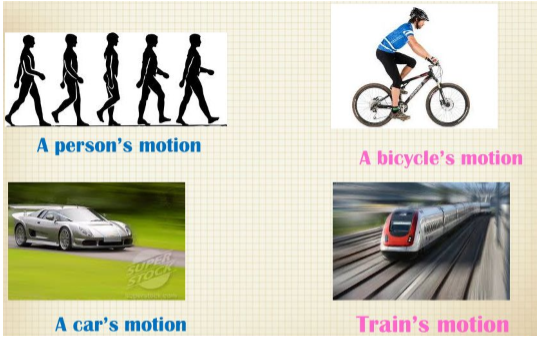Figure 1: Examples of Motion

Slow and Fast Motion

If one object covers a particular distance in less time and another object covers the same distance in more time then the first object is said to be moving slowly while the second object is said to be moving faster.

The Speed of an object

The distance travelled by an object in unit time is called its Speed.

Types of Speed:

Uniform Speed - When the object travels a fixed distance same time gaps, it is said to have a uniform speed.

Non-uniform speed - When an object covers different distances in different time gaps, it is said to have a non-uniform speed.

Average speed - The total distance travelled by an object divided by the total time taken by the object is called its average speed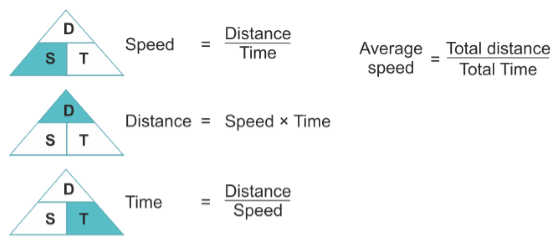Figure 2: Finding Speed, Time and Distance

Measuring Time

There are many events in nature that repeat after a time interval:

Morning – The rising of the sun

Day and Night – The time between the sunrise and sunset

Month – The time between two new moons

Year – The time the earth takes to complete its one revolution around the sun

Time measuring devices or clocks - Clocks use the concept of periodic motion to measure time. It means that it uses motion that repeats itself in equal amounts of time. There are different types of time measuring devices.

Sundial – It uses the position of the sun to depict timeSand Clock (hourglass) – It uses sand to measure time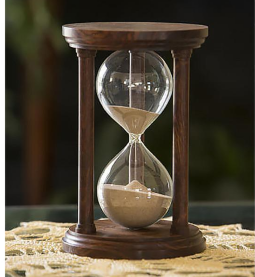Water Clock – It uses water to measure time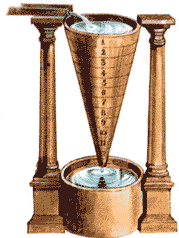Pendulum Clock – It uses a pendulum to measure time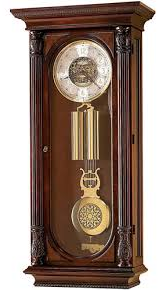Quartz Clocks – They have an electric circuit that works with the help of cells. They provide accurate time.Periodic Motion of a Simple Pendulum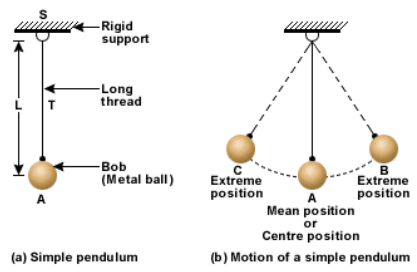Figure 8: Simple Pendulum

A simple pendulum contains a Bob. It is a metallic ball or a stone which is suspended from a rigid stand with the help of a thread.

Oscillatory motion - The to and fro motion of the pendulum is called as Oscillatory Motion. The bob of the pendulum does move from the centre (mean position) of the pendulum to its extreme positions on the other side.

Oscillation - When the bob moves from its centre (mean position) to its extreme ends it is said to complete one oscillation.

Time Period of a pendulum - The time taken by the pendulum bob to complete one oscillation is called its Time Period.

Units to Measure Time Speed

Time

Second (s)

Minutes (min)

Hours (h)

Speed = Distance/time

Meter/Second (m/s)

Meter/minute (m/min)

Kilometer/hour (km/h)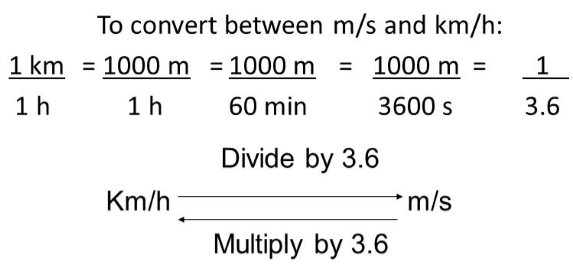Figure 9: Conversion between km/hr and m/s

Speedometer - It is a device which is used in vehicles such as cars and trucks which measures the speed in kilometer per hour.

Odometer - It is a device which measures the distance travelled by a vehicle in meters or kilometers.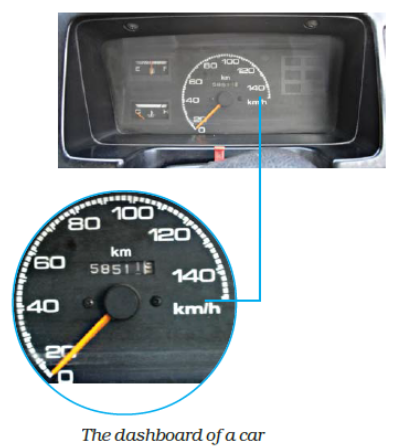Figure 10: Measure of Distance and Speed of a car

Distance-time Graph

A graph which represents the distance travelled by an object with respect to time is called a distance-time graph.

Making a distance-time graph:

1. Mark the x-axis and y-axis and divide them in equal quantities.Figure 11: Take the first quadrant

2. Choose one scale to represent distance (for example, x-axis to represent distance where 1 km = 1 cm) and the other to represent time (for example, y-axis to represent the time where 1 min = 1 cm).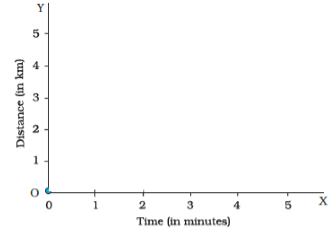Figure 12: Choosing the scale

3. Mark the values of time and distance in the graph.
4. Mark the set of values of time taken and distance covered in that time by the object in the graph. For example, if 1 km is covered in 1 minute then mark 1 unit on both the x-axis and y-axis.Figure 13: Marking the values for time and distance

5. Now draw lines parallel to x-axis and y-axis at the points that you have marked.
6. Mark the points where these lines intersect on the graph. These points show the position of the moving object.
7. Now join all the points of intersection and obtain a straight-line graph.
8. This is the distance-time graph of a moving object.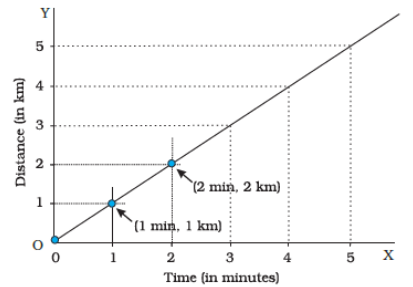Figure 14: Obtaining a straight line graph

The shape of the distance-time graph can be the following:

Shape of Graph
Interpretation

Straight line

The object has a uniform or constant speed

Parallel to time-axis

It is a stationary object

Curve shape

The object has a non-uniform speedFigure 15 Distance-time Graphs

To find the speed of the distance-time graph

Speed = distance/time = (final position of object – initial position of object)/time taken by object

Also, the speed of the distance-time graph can be calculated by the Slope of a graph. The steeper the slope of the graph, the more is the speed of the object. For example, in the graph given below object A has a steeper slope. This means that object A is moving at a higher speed than object B.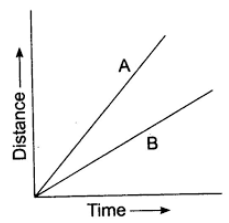Figure 16 Distance-time graph of two objects
<!--td {border: 1px solid #ccc;}br {mso-data-placement:same-cell;}-->

```### Course Features

• 728 Video Lectures
• Revision Notes
• Previous Year Papers
• Mind Map
• Study Planner
• NCERT Solutions
• Discussion Forum
• Test paper with Video Solution